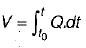Courses

# Test: Runoff - 1

## 10 Questions MCQ Test GATE Civil Engineering (CE) 2022 Mock Test Series | Test: Runoff - 1

Description
This mock test of Test: Runoff - 1 for Civil Engineering (CE) helps you for every Civil Engineering (CE) entrance exam. This contains 10 Multiple Choice Questions for Civil Engineering (CE) Test: Runoff - 1 (mcq) to study with solutions a complete question bank. The solved questions answers in this Test: Runoff - 1 quiz give you a good mix of easy questions and tough questions. Civil Engineering (CE) students definitely take this Test: Runoff - 1 exercise for a better result in the exam. You can find other Test: Runoff - 1 extra questions, long questions & short questions for Civil Engineering (CE) on EduRev as well by searching above.
QUESTION: 1

### Direct runoff is made up of

Solution:

Direct runoff is that part of runoff which enters the stream immediately after the precipitation. It includes surface runoff, prompt interflow and precipitation on the channel surface.

QUESTION: 2

Solution:
QUESTION: 3

### The term base flow denotes

Solution:

The delayed flow that reaches a stream essentially as groundwater flow is called base flow.

QUESTION: 4

Virgin flow is

Solution:

A stream flow unaffected by works of man, such as structures for storage and diversion on a stream is called virgin flow.

QUESTION: 5

An ephemeral stream

Solution:
QUESTION: 6

An intermittent stream

Solution:

An intermittent stream has limited contribution from the groundwater. During the wet season the water table is above the stream bed and there is a contribution of the base flow to the stream flow.

QUESTION: 7

The flow-duration curve is a plot of

Solution:

A flow-duration curve of a stream is a plot of discharge against the per cent of time the flow was equalled or exceeded. This curve is also known as discharge frequency curve.

QUESTION: 8

In a flow-mass curve study the demand line drawn from a ridge in the curve did not interest the mass curve again. This represents that

Solution:
QUESTION: 9

If in a flow-mass curve, a demand line drawn tangent to the lowest point in a valley of the cure does not intersect the mass curve at an earlier time period, it represents that

Solution:
QUESTION: 10

The flow-mass curve is an integral curve of

Solution:

The flow mass curve is a plot of the cumulative discharge volume against time plotted in chronological order. The ordinate of the mass curve, V at any time t is,Since the hydrograph is a plot of Q vs t, it is easy that the flow mass curve is an integral curve of the hydrograph.